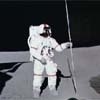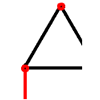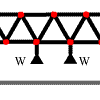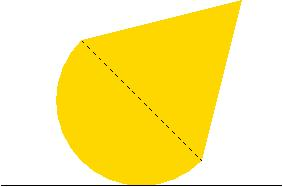You may also likeLunar Leaper

Gravity on the Moon is about 1/6th that on the Earth. A pole-vaulter 2 metres tall can clear a 5 metres pole on the Earth. How high a pole could he clear on the Moon?Bridge Builder

In this short problem we investigate the tensions and compressions in a framework made from springs and ropes.More Bridge Building

Which parts of these framework bridges are in tension and which parts are in compression?

Wobbler

Age 16 to 18 Challenge Level:A solid hemisphere of radius $a$ and a solid right circular cone of height $h$ and base radius $a$ are made from the same uniform material and joined together with their circular faces in contact. Find the position of the centre of gravity of the whole body.

If the body is placed on a horizontal table with its hemispherical surface in contact with the table in what position will it come to rest if (i) $h=a$ and (ii) $h=2a$?

(iii) If the body always rests in equilibrium when it is placed on a horizontal table with a point of the hemispherical surface in contact with the table find $h$ in terms of $a$ and the angle of the cone in this case.

(iv) In what position does the body rest in equilibrium for other angles of the cone (other values of $h/a$) and when will the equilibrium be stable?